# Test: Electricity - 1

## 10 Questions MCQ Test Science & Technology for UPSC CSE | Test: Electricity - 1

Description
Attempt Test: Electricity - 1 | 10 questions in 10 minutes | Mock test for Class 10 preparation | Free important questions MCQ to study Science & Technology for UPSC CSE for Class 10 Exam | Download free PDF with solutions
QUESTION: 1

### All elements of an electric circuit are connected in series except :

Solution:

In an electric circuit all other elements are connected in series but a voltmeter is connected in parallel.

QUESTION: 2

### The least resistance obtained by using 2Ω , 4Ω, 1Ω and 100Ω is

Solution:

In parallel combination, the equivalent resistance is Smaller than the least resistance used in the circuit.

QUESTION: 3

### A resistance wire is stretched so as to double its length. Its new resistivity will have a magnitude

Solution:

When a wire is stretched, its resistivity remains unchanged. It is because resistivity of a conductor depends only on its material and is independent of its length or cross- section area.

QUESTION: 4

Two devices are connected between two points say A and B in parallel. The physical quantity that will remain the same between the two points is

Solution:

In parallel combination voltage remains same across two points.

QUESTION: 5

The resistance of an alloy :

Solution:

The resistance of an alloy increases with increase in temperature.

QUESTION: 6

To get 2Ω resistance using only 6Ω resistors, the number of them required is

Solution:

Three resistors of 2Ω is required to get 6Ω because resultant is more than individual so they all must be connected in parallel.

QUESTION: 7

Three resistors of 1Ω, 2 Ω and 3 Ω are connected in series with a battery of 12 V as shown in figure. Values of potential differences V1, V2, V3 across the three resistors have respective values :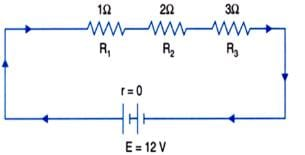Solution:

In series grouping current I is same throughout and potential differences are directly proportional to respective resistances.
In given circuit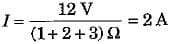∴ V1 = IR= 2 A x 1Ω = 2V,

V2 = IR2 = 2 A x 2 Ω = 4 V and

V3 = IR3 = 2 A x 3 Ω = 6 V.

QUESTION: 8

The resistance whose V - I graph is given below is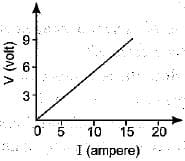Solution:

Resistance = slope line of V-I graph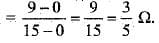QUESTION: 9

The diagram shows a network of four resistors which is connected to an electric source. Identify the resistors which are connected in series in this network :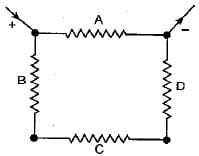Solution:

In the circuit shown, the resistors B, C and D are connected in series.

QUESTION: 10

A boy records that 4000 joule of work is required to transfer 10 coulomb of charge between two points of a resistor of 50Ω. The current passing through it is

Solution:

Work done in transferring the charge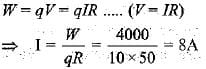Use Code STAYHOME200 and get INR 200 additional OFF Use Coupon Code